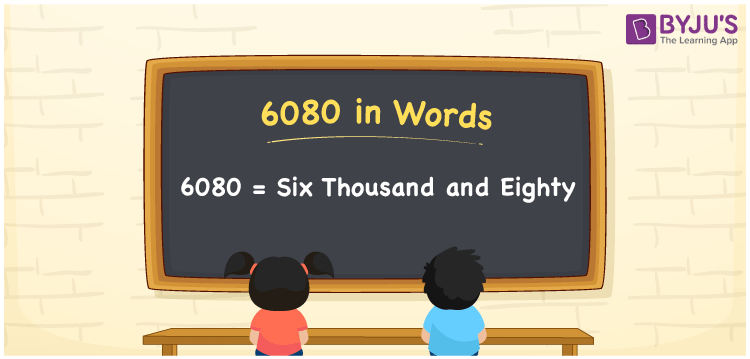# 6080 in Words

6080 in words is written as Six thousand eighty. In both the International System of Numerals and the Indian System of Numerals, 6080 is written as Six thousand eighty. The number 6080 is a Cardinal Number as it represents some quantity. For example, “the dress costs 6080 rupees”.

 6080 in Words Six thousand eighty Six thousand eighty in Number 6080

## 6080 in English Words

6080 in English words is read as “Six thousand eighty.”## How to Write 6080 in Words?

To write 6080 in words, we shall use the place value chart. In the place value chart, put 6 in the thousands, 0 in the hundreds, 8 in the tens, and 0 in the ones, respectively. Let us make a place value chart to write the number 6080 in words.

 Thousands Hundreds Tens Ones 6 0 8 0

Thus, we can write the expanded form as

6 × Thousand + 0 × Hundred + 8 × Ten + 0 × One

= 6 × 1000 + 0 × 100 + 8 × 10 + 0 × 1

= 6000 + 0 + 80 + 0

= 6080

= Six thousand eighty.

6080 is a natural number which is the successor of 6079 and the predecessor of 6081.

6080 in words – Six thousand eighty

• Is 6080 an odd number? – No
• Is 6080 an even number? – Yes
• Is 6080 a perfect square number? – No
• Is 6080 a perfect cube number? – No
• Is 6080 a prime number? – No
• Is 6080 a composite number? – Yes

## Frequently Asked Questions on 6080 in Words

Q1

### How to write 6080 in words?

6080 in words is written as Six thousand eighty.
Q2

### How to write 6080 in words in the International and Indian System of Numerals?

In both, the system of numerals, 6080 in words, is written as Six thousand eighty.
Q3

### How to write 6080 in a place value chart?

In the place value chart, write 6 in the thousands, 0 in the hundreds, 8 in the tens, and 0 in the ones, respectively.